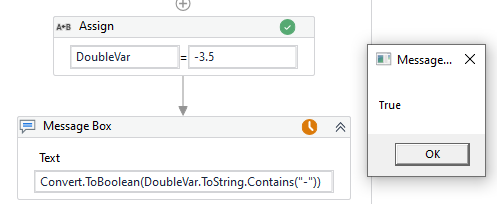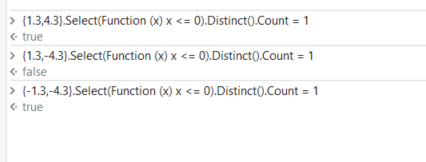# If two variables are positive or negative

Hi,

I have two double variables. I want to check if both are positive or negative. How can this be accomplish?

Hi @RPA_Path,

You could try:
`DoubleVar.ToString.Contains("-")` and if it does then it’s a negative

Edit: If you used this in an if statement it’d give a True or False:If you only are interested in knowing whether both are negative or positive, then you can multiply them.
If the result is positive, then both is positive or both is negative.
If the result is negative, then one is positive and one is negative.
If the result is zero, then one or both is zero.

How would you check if two variables contains (“-”)?

Hi @RPA_Path,

In an if statement:
`DoubleVar1.ToString.Contains("-") And DoubleVar2.ToString.Contains("-")`

If both items contain “-”, then it will return True. In the Else, you could then put:
`DoubleVar1.ToString.Contains("-") Or DoubleVar2.ToString.Contains("-")`

And this will return True if one contains a hyphen and the other doesn’t. It will return false if both are positive and don’t contain hyphens

1 Like

This topic was automatically closed 3 days after the last reply. New replies are no longer allowed.